Back[TOC]

AP 各学科「速成」笔记导览

# Chemical Bonds 化学键

• Chemical Bonds is Attraction ## Metallic Bonds 金属键

• 金属有很多的自由电子，我们称之为 Mobile Electrons or Delocalized Electron (Sea of Electrons)

• 金属键就是金属阳离子与这些自由电子之间的吸引力（Attraction）

• 这些自由电子也很好的解释了为什么 金属是电和热的良好导体### Properties of Metals 金属的性质

• 金属的延展性很好（Malleable and Ductile），层与层之间可以轻易滑动
• 这是因为形成金属键电子海中的电子是可移动的离域电子（Delocalized Electrons），这些离域电子可以在整个巨大的金属晶格中自由移动，所以当一层金属离子在另一层上滑动时，电子也可以移动，使整个结构粘合在一起。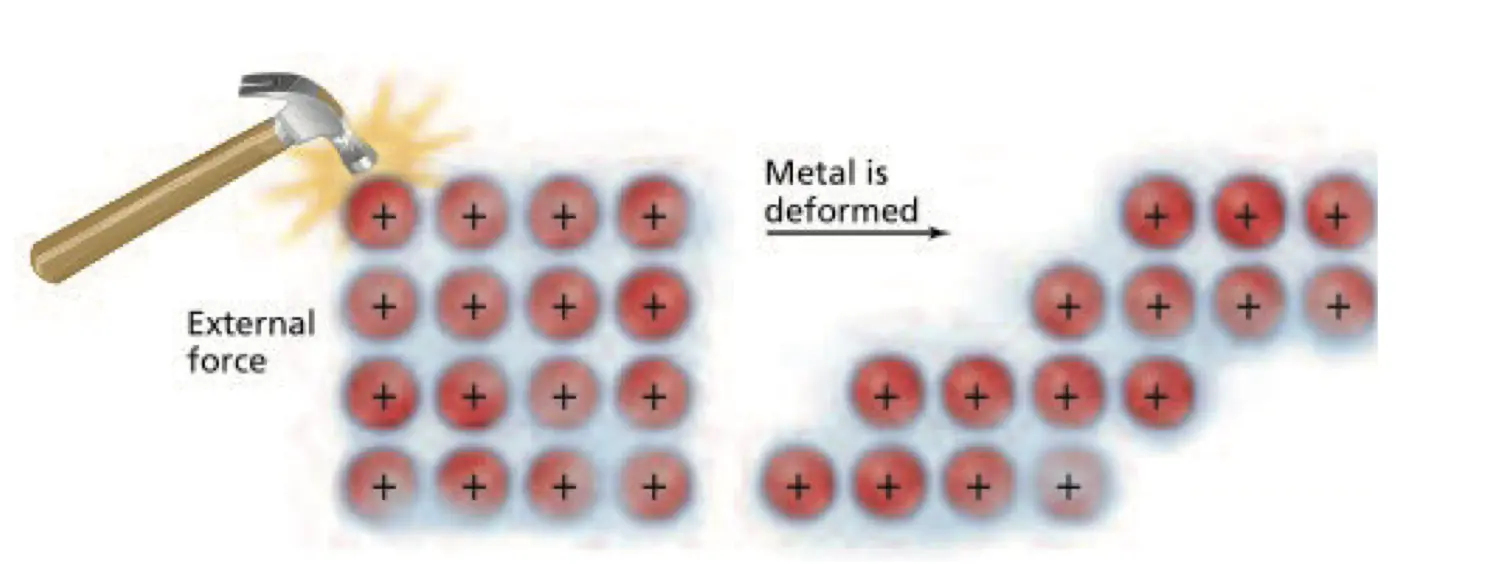• 随着金属键的强度增加，金属的融沸点就会增加。
• 金属键的强度由以下两点决定
• 原子半径
• 自由电子的强度
• 而由于自由电子强度受影响的因素太多，故我们只考虑第一主族金属键强度的变化，也就是随着原子半径增加，金属键强度减少（吸引力减少）
• 例如：$$\ce{Na}$$ 熔点 $$\pu{882.9 ^\circ C}$$$$\ce{K}$$ 熔点 $$\pu{774 ^\circ C}$$

### Alloy

• 填隙式合金（Interstitial Alloy），原子半径差异大
• 硬，降低延展性
• 取代式合金 （Substitutional Alloy），原子半径差异小
• 因为破坏了晶格结构，相对纯金属延展性降低，但是相对填隙式合金更好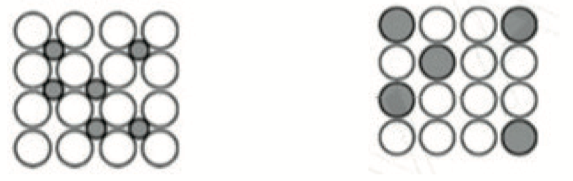• AP 2014: B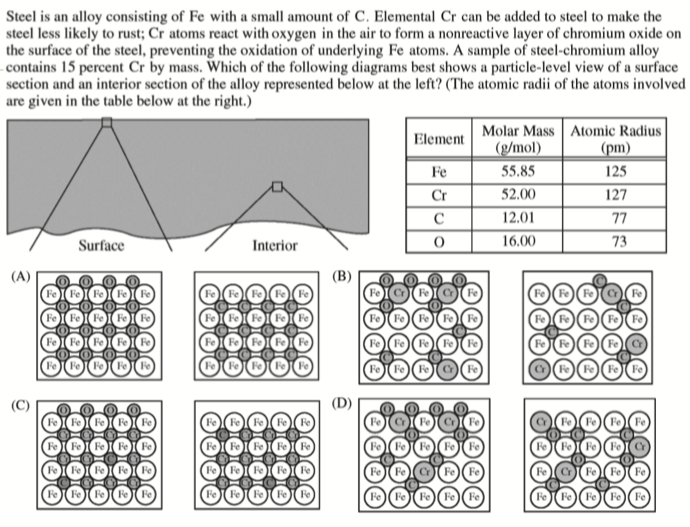• $$R_\ce{Cr} \thickapprox R_\ce{Fe}$$ 所以排除 AC，再根据题意，右图应为金属内部图，而题意指出铬是为了防止金属氧化，所以内部不应有 $$\ce{O}$$，故选 B

## Ionic Bonds 离子键

• 一般形成与金属与非金属之间，离子键的本质是正负离子的吸引，形成的原因是电子的转移

$\ce{Na+[Cl-]}$

• $$\ce{NH+4Cl-}$$ 便是非金属与非金属之间的离子键

• 离子化合物固态不导电，熔融态（液态）可导电

• 这是因为熔融态离子化合物中的离子才能自由移动
• 一般而言，离子化合物相对较硬且有更高的熔沸点，并且因为正负电子的排斥效应，会使得离子化合物相对更脆

• Practice: A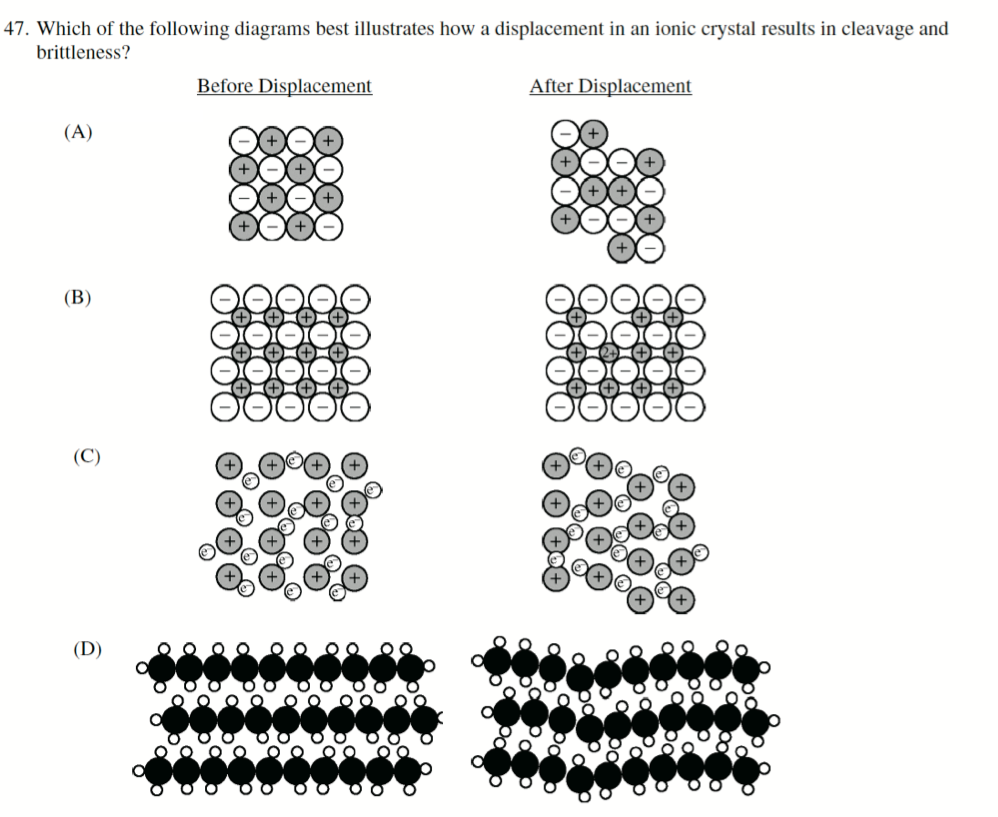### Lattice Energy 晶格能

• 晶格能（Lattice energy），将阴阳离子分开的所需的能量即为晶格能

• 因为发生相变需要破坏离子键和晶格结构，也就是说，离子键越强化合物熔沸点越高

• 而离子键的强度大部分受到库仑定律的影响

$F = \frac{q_1 q_2 \; \text{(Charge Magnitude)}}{r \; \text{(Distance between Ions)}}$

• Practice: B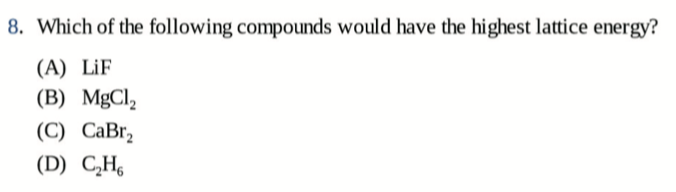• Practice： A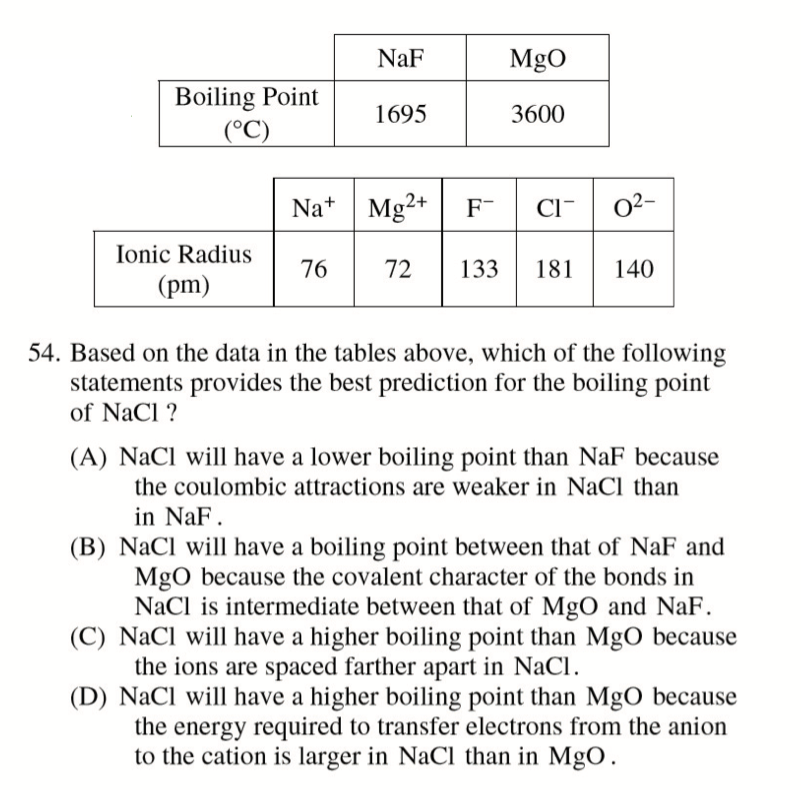## Covalent Bonds 共价键

• 共价键，Covalent Bonds，两个原子共用电子，即为共价键
• 与离子键的区别在于，离子键发生了电子的转移，而共价键是共享电子

### Polarity of Covalent Bonds 共价键的极性

• 非极性共价键（Nonpolar Covalent Bonds），即共用电子的两个原子获得电子的能力（电负性）基本一致，e.g. $$\ce{Cl_2}$$
• 极性共价键（Polar Covalent Bonds），即共用电子的两个原子获得电子的能力（电负性）差异较大，e.g. $$\ce{HCl}$$
• 参阅 Electronnegativity 电负性

$\ce{H(\delta^+) Cl(\delta^-)}$

• 分子内都是极性键，不一定分子就是极性分子，e.g. $$\ce{CO2, CCl_4}$$，分子极性主要取决于空间构型

• 偶极矩（Dipole Moment）是正、负电荷中心间的距离和电荷中心所带电量的乘积，它是一个矢量，方向规定为从正电中心指向负电中心

$\mu = \delta \vec{d}$

• Practice: A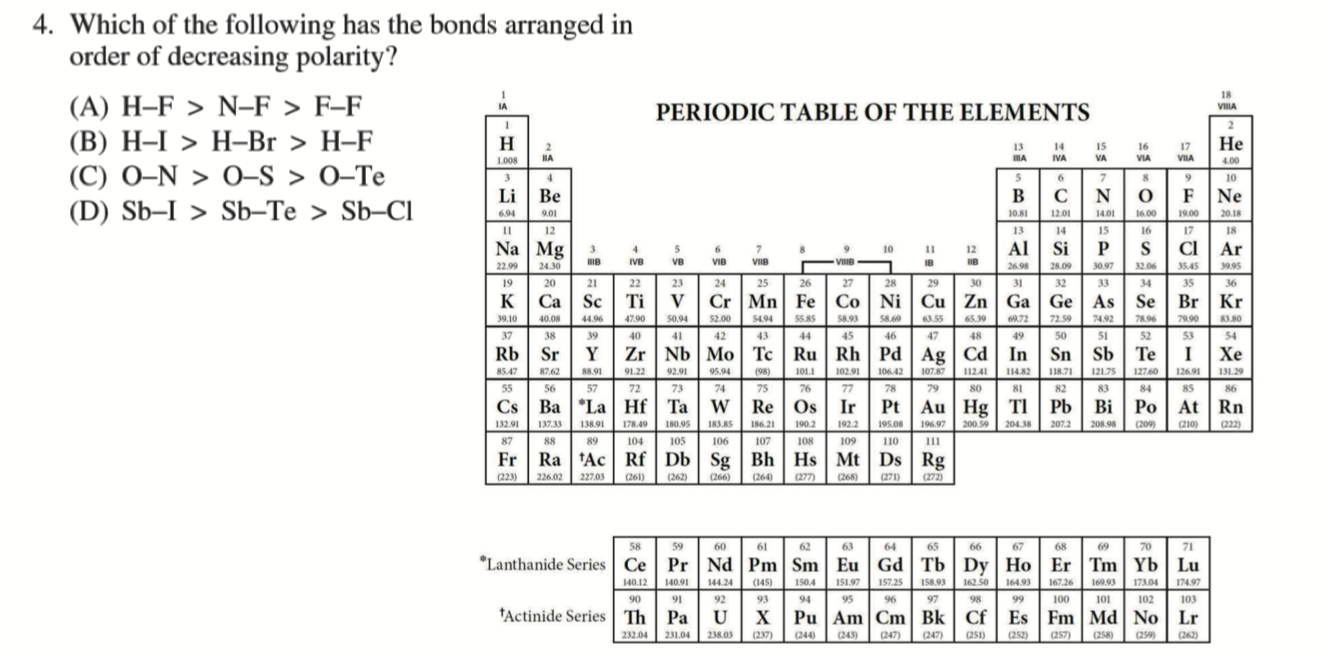### Bond Length and Bond Energy 键长与键能

• 键长（Bond Length），即为两个原子半径之和

• 键能（Bond Energy），即为破坏这根键所需要的能量

• 一般而言，键能符合如下规律

\begin{aligned} \ce{H&-H} \; \text{(Small)} \\ \ce{O&\bond{2}O} \\ \ce{N&\bond{3}N} \; \text{(Big)} \end{aligned}

• 键长则符合如下规律

\begin{aligned} \ce{C&-C} \; \text{(Small)} \\ \ce{C&\bond{2}C} \\ \ce{C&\bond{3}C} \; \text{(Long)} \end{aligned}

### Formation of Covalent Bonds 共价键的形成

• 在图示最低点，纵座标与上方势能零点的差值为键能，横座标为键长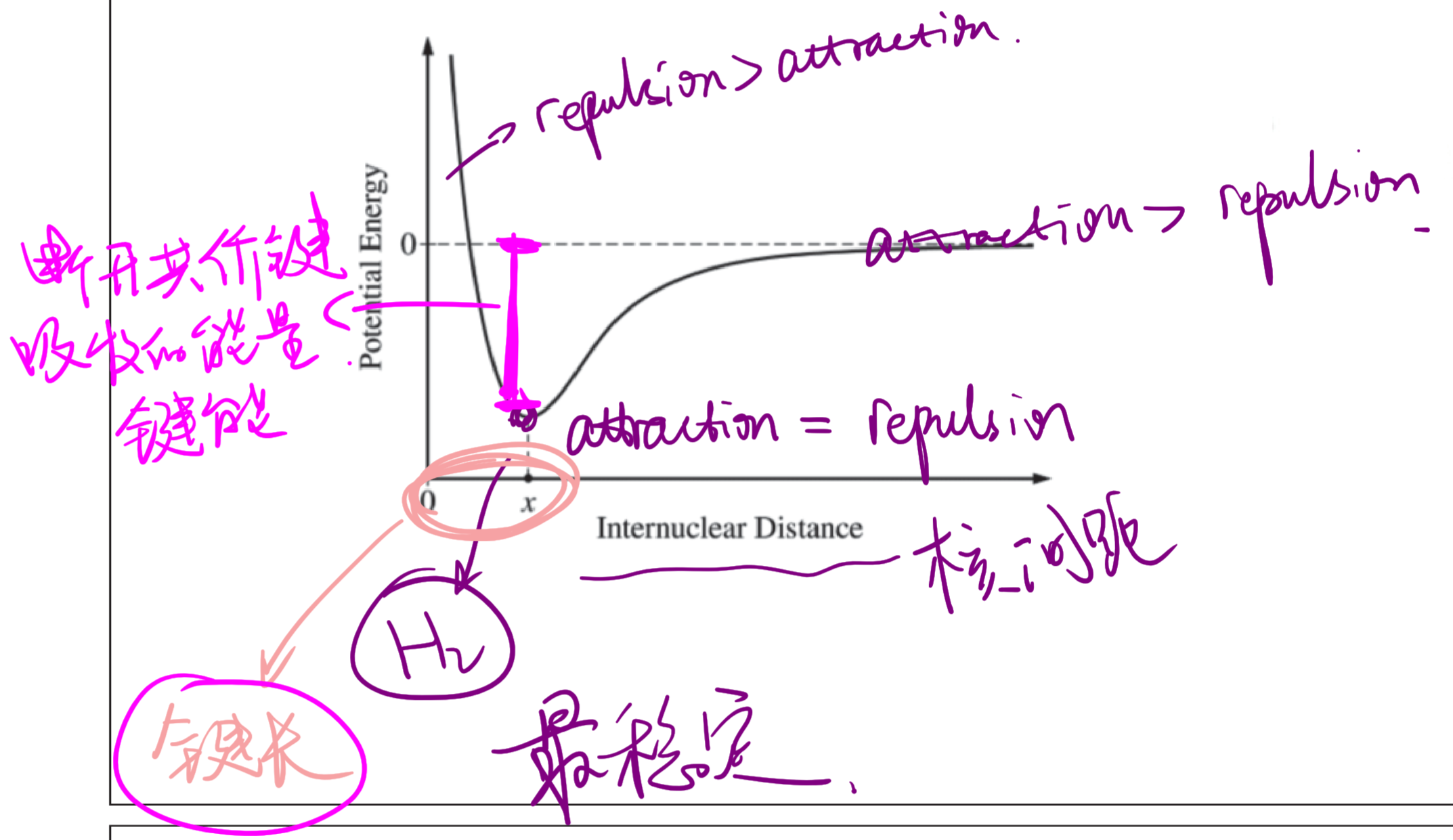• Practice: A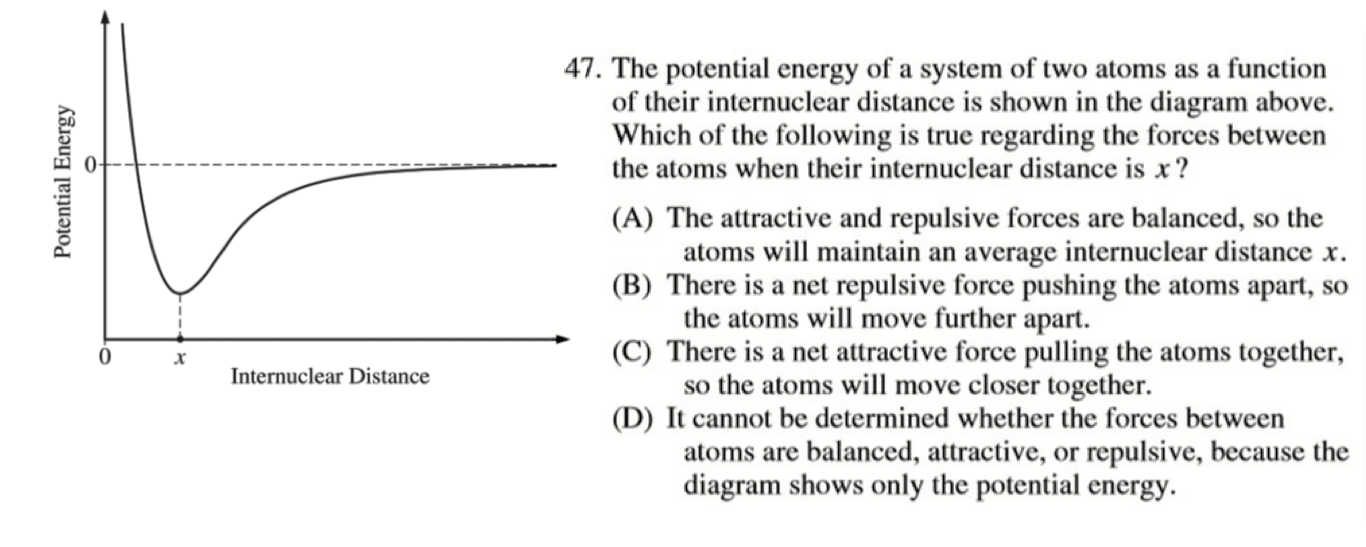• AP 2014: A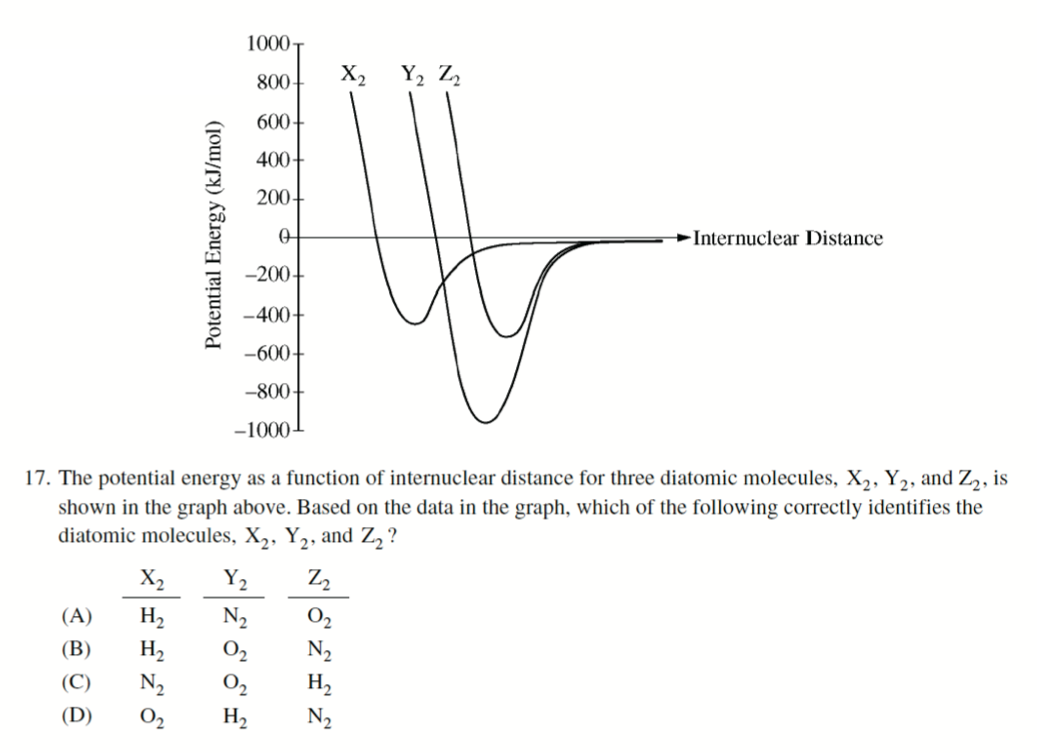• $$\ce{H-H}, \ce{N\bond{3}N}, \ce{O\bond{2}O}$$
• 注意此题不能比较键长，键长只能同类比较

### Lewis Structure Diagram 路易斯结构图

• 路易斯结构图（Lewis Structure Diagram），展现的是最外层电子的排布，一般情况下需要符合八隅体规则（Octet Rule）
• 成键电子对（Bonding Pair）
• 孤对电子（Lone Pair）

#### How to Draw Lewis Structure Diagram 如何画路易斯结构图

• 一般的，我们认为第七主族的元素倾向于形成一根共价键，第六主族元素倾向于形成两根，以此类推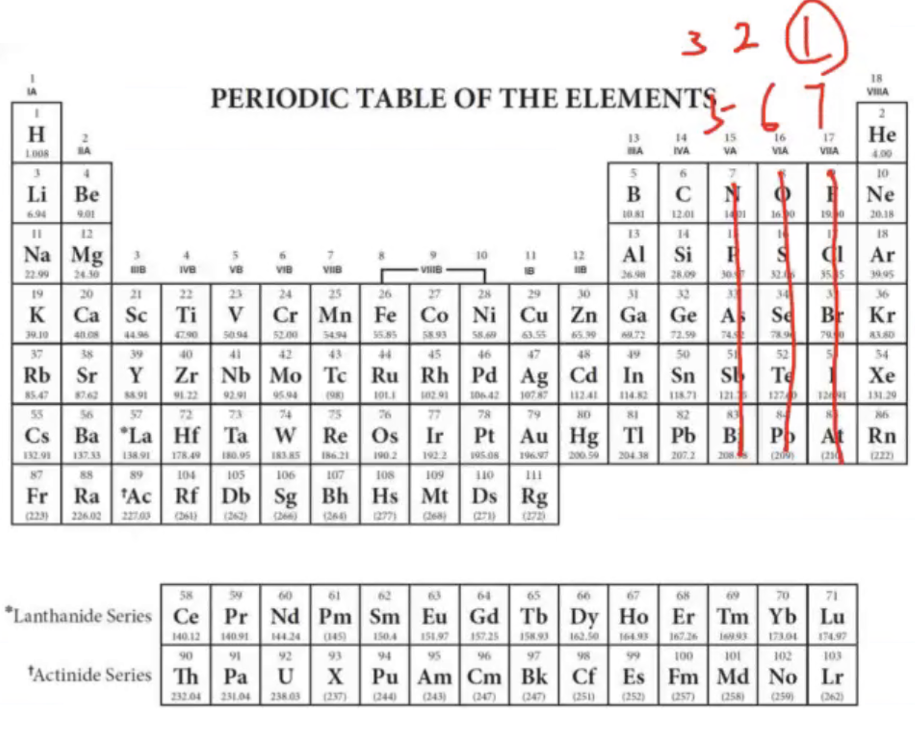• 对于更一般的情况，我们遵循以下步骤
• 确定中心原子（有碳选碳，没碳选电负性小的）
• 计算每个原子的价电子数量
• 让周围的原子按顺序尽量满足最外层八电子/二电子
• 当中心原子已经八电子时，考虑使用配位键让剩下的周围原子满足最外层八点子（下一小结的特例除外）
• Practice: $$\ce{H-O-N\bond{2}O}$$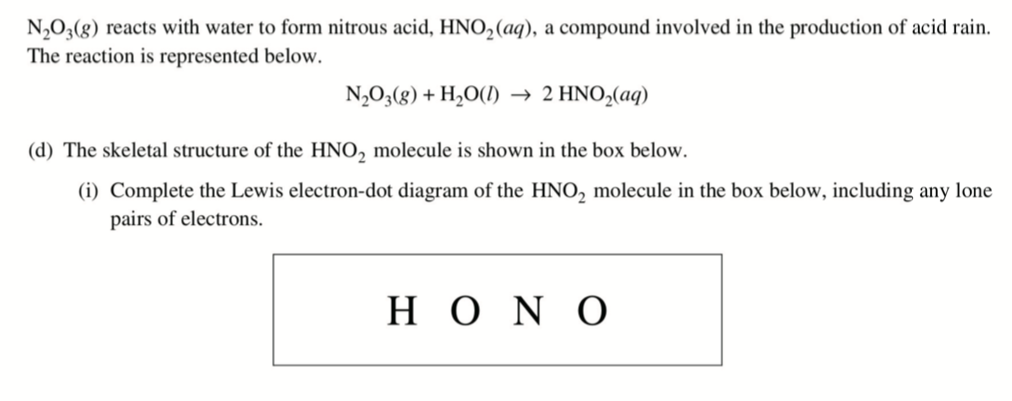• 注意，不可以是 $$\ce{H-O\bond{2}N-O}$$$$\ce{O}$$ 只能伸出两根键

#### Resonance 共振

• 共振结构意味着有多种路易斯结构画法，如图所示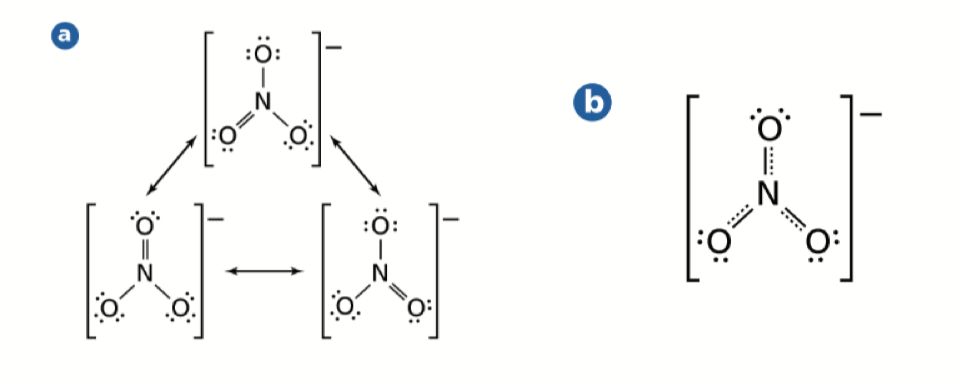• 但共振的本质是电子在不同地方同时存在，可以认为是一种运动，我们也称这种键为离域 $$\pi$$ 键（Delocalized $$\pi$$ Bond）（苯环中的圆便是共振结构）

#### Exception of Lewis 特例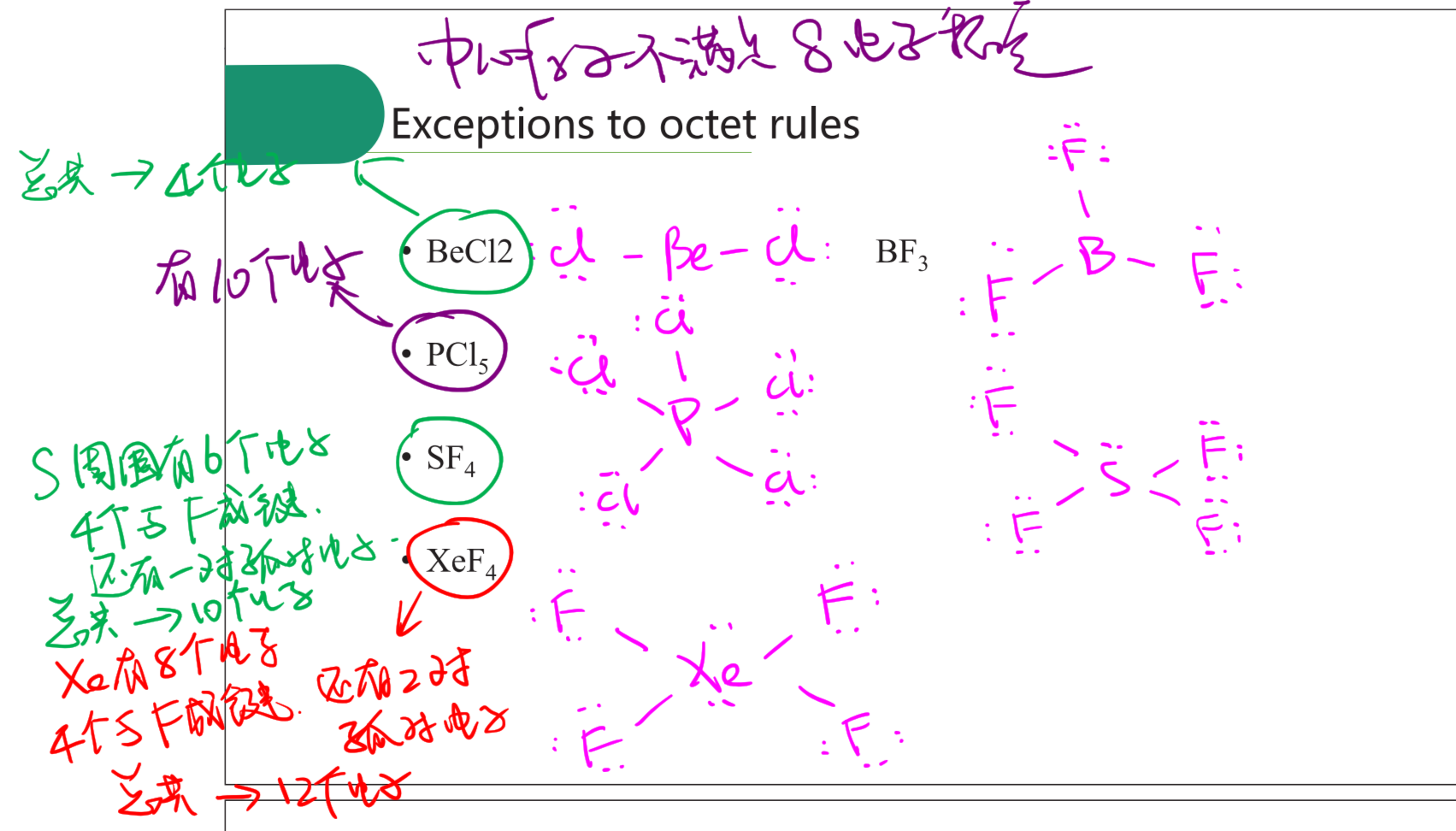### VSEPR Model

• 价层电子对排斥模型（Valence Shell Electron Pair Repulsion Model）

• 电子域（Electron Domain），成键域（Bonding Domain），非成键域（Nonbonding Domain）

• $$\text{Lone Pair - Lone Pair} > \text{Lone Pair - Bonding Pair} > \text{Bonding Pair - Bonding Pair}$$

Molecular TypeElectron GeometryMolecular GeometryApproximate Bond AnglesFigureExample
$$\ce{AX2E_n}$$LinearLinear$$180^\circ$$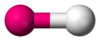$$\ce{O_2}$$
$$\ce{AX2E0}$$LinearLinear$$180^\circ$$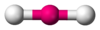$$\ce{BeCl2, HgCl2, CO2}$$
$$\ce{AX3E0}$$Trigonal PlannarTrigonal Plannar$$120^\circ$$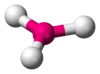$$\ce{BF3, CO3^2-, NO3^-, SO3}$$
$$\ce{AX2E1}$$Bent$$109.5^\circ$$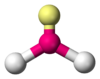$$\ce{NO2^-, SO2, O3}$$
$$\ce{AX4E0}$$Tetrahedral$$<109.5^\circ$$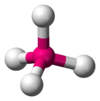$$\ce{CH4, PO4^3-, SO4^2-, ClO4^-}$$
$$\ce{AX3E1}$$Trigonal Pyramidal$$<109.5^\circ$$$$\ce{NH3, PCl3}$$
$$\ce{AX2E2}$$Bent$$<<109.5^\circ$$$$\ce{H2O, OF2}$$
$$\ce{AX5E0}$$Trigonal BipyramidalTrigonal Bipyramidal$$120^\circ$$, $$90^\circ$$$$\ce{PCl5}$$
$$\ce{AX4E1}$$Seesaw$$<120^\circ$$, $$<90^\circ$$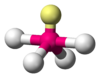$$\ce{SF4}$$
$$\ce{AX3E2}$$T-shaped$$<90^\circ$$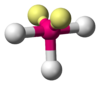$$\ce{ClF3, BrF3}$$
$$\ce{AX2E3}$$Linear$$180^\circ$$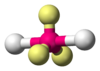$$\ce{XeF2, I3^-}$$
$$\ce{AX6E0}$$OctahedralOctahedral$$90^\circ$$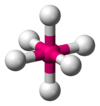$$\ce{SF6}$$
$$\ce{AX5E1}$$Square Pyramidal$$<90^\circ$$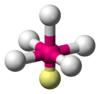$$\ce{ClF5, BrF5}$$
$$\ce{AX4E2}$$Square Planar$$90^\circ$$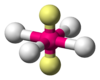$$\ce{XeF4}$$

### Molecular Polarity 分子极性

• 当没有 Lone Pair，且周围原子都是一样时认为所有的力可以被相互抵消（cancel out），除了 $$\ce{AX_2E_3} ,\; \ce{AX_4E_2}$$• 在 FRQ 中，应回答 “The $$\ce{XXX}$$ molecule is nonpolar because all of the bond dipoles in the molecule have been cancelled out.”

• Practice: D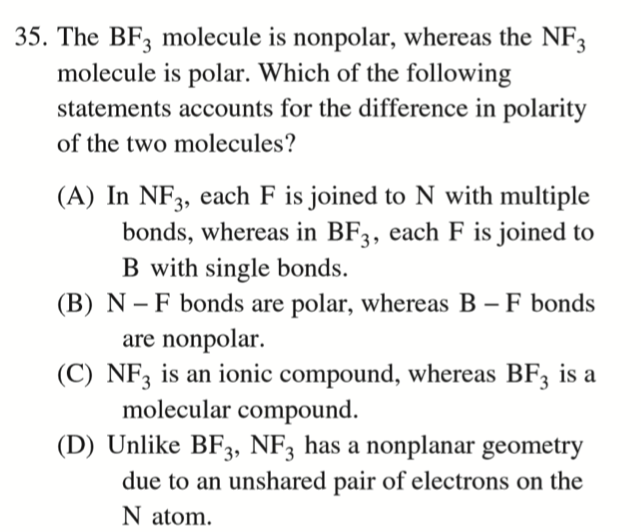• $$\ce{BF3}$$ 的构型为 $$\ce{AX3}$$, 无 Lone Pair 所以是 Nonpolar，但是 $$\ce{NF3}$$ 的构型为 $$AX3E1$$，有 Lone Pair 且不是 $$\ce{AX_2E_3} ,\; \ce{AX_4E_2}$$，可以在平面上抵消，所以是 Polar

### Hybridization 杂化

$$\ce{CH4}$$ 的结构图上，我们认为 $$\ce{C}$$ 对四个 $$\ce{H}$$ 形成的共价键是等价的，这需要 $$\ce{C}$$ 外层的 4 个电子 $$\ce{2s^2 2p^2}$$，但同时显然这两个不同轨道上的电子形成的键不会相同，所以杂化理论孕育而生用于解释这种现象。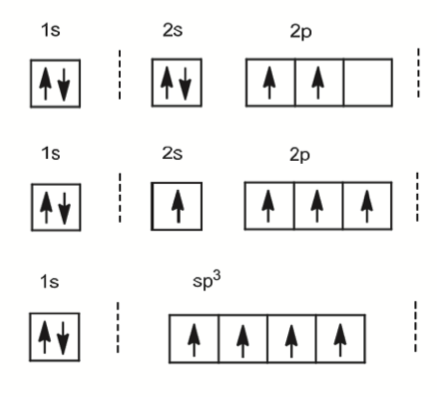$$\ce{sp^2 sp}$$ 杂化同理，轨道总数保持相同。

• Practice: $$\ce{H-O-N\bond{2}O}$$$$\ce{N}$$ 的杂化方式是？ ── $$3 - 1 = \ce{sp^2}$$ 杂化

• Practice: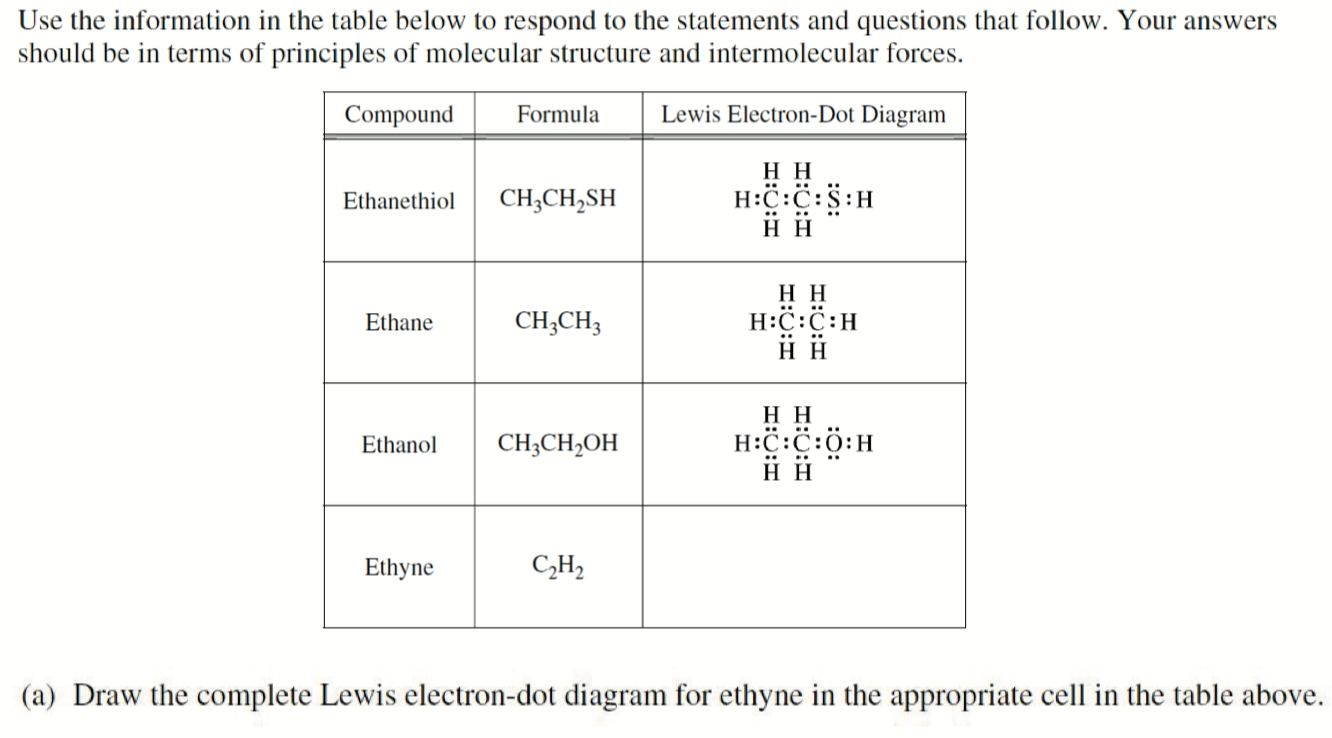1. Draw the complete Lewis electron-dot diagram for ethyne in the appropriate cell in the table above.

$$\ce{H-C\bond{3}C-H}$$，首先确定两个 $$\ce{C}$$ 为中心，且 $$\ce{C}$$ 至少伸出 4 键，而 $$\ce{H}$$ 最多 1 键

1. Which of the four molecules contains the shortest carbon-to-carbon bond? Explain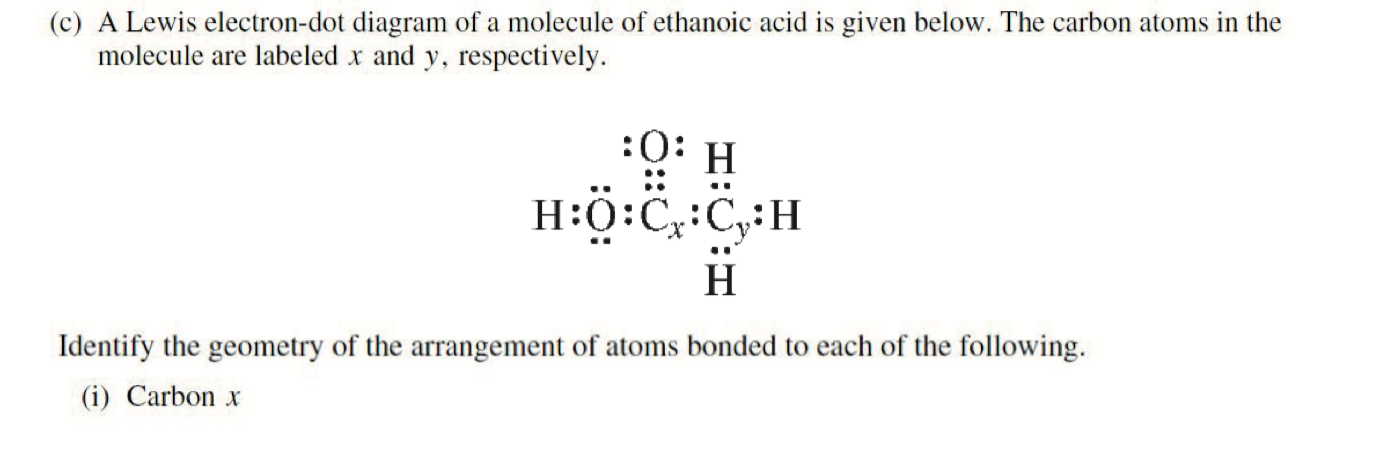• $$\ce{C_x}$$ : $$\ce{AX3}$$ Trigonal Planar

• $$\ce{C_y}$$ : $$\ce{AX4}$$ Tetrahedral

## Bond Order, Resonance and Formal Charge 键级, 共振与形式电荷

### Sigma & Pi Bond

• Sigma $$\sigma$$ Bonds: 「头碰头」方式重叠，重叠面积比较大，键比较稳定 Linear Overlap (End-on)
• Pi $$Bonds: 「肩并肩」方式重叠，重叠面积较小，键不那么稳定 Sideway Overlap 有言道「沿轴头碰头，平行肩并肩」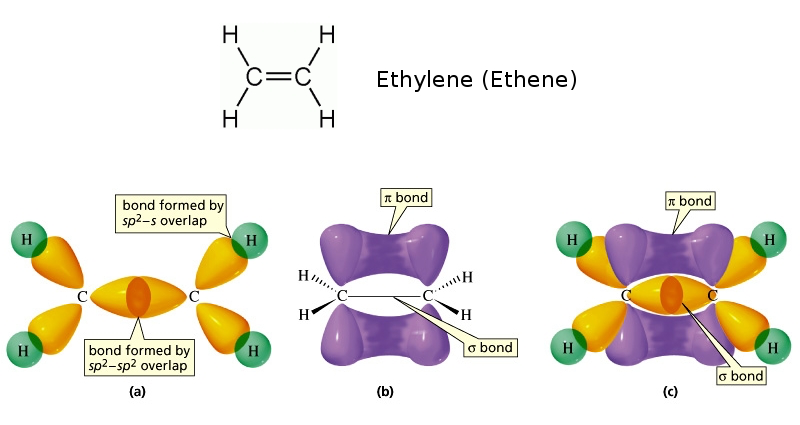Bond Type:SingleDoubleTriple Bond Designation:$$\sigma$$$$\sigma$$ and $$\pi$$$$\sigma$$ and $$2\pi$$ Bond Order:122 Bond Length:LongestIntermediateShortest Bond Energy:LeastIntermediateGreatest • Bond Order, 键级 在 AP 中可以简单理解为「键的强度」 ### Resonance and Bond Order 共振与键级 • 对于共振结构的化合物，其共振本质上是电子在不同位置上做运动形成「离域$$ 键」，可以认为其键的强度和各种性质均为正常键的 $$\frac{m}{n}$$，其中 $$m$$ 为总键数（固定键 + 可变键），$$n$$ 为固定键• 所以对于上图，$$\ce{[NO3]-}$$$$\ce{N\bond{~-}O}$$ 键长，应介于 $$\ce{N\bond{-}O}$$$$\ce{N\bond{2}O}$$ 之间
• 其中固定键为 $$3$$，可变键为 $$1$$，键级为 $$\frac{3+1}{3} = \frac{4}{3}$$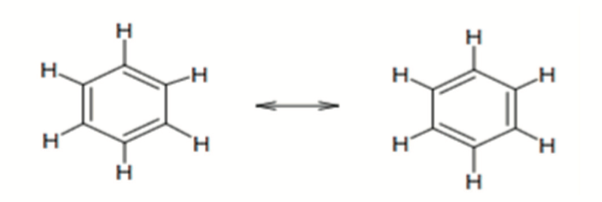• 苯环 $$\ce{C6H6}$$，其中固定键为 $$6$$，可变键 $$3$$，键级为 $$\frac{6 + 3}{3} = \frac{9}{3} = 1.5$$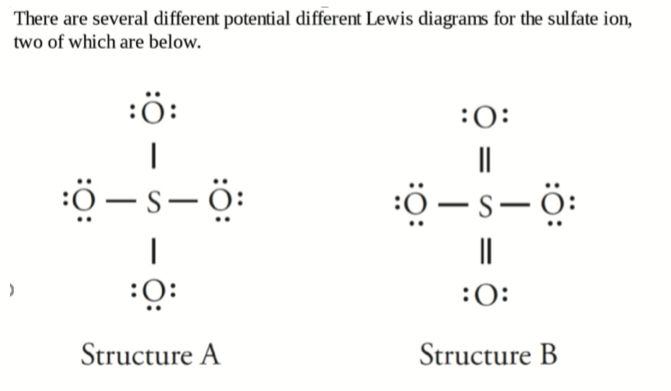• 固定键为 $$4$$，可变键为 $$2$$，键级为 $$\frac{4 + 2}{4} = \frac{6}{4} = 1.5$$

• Practice: D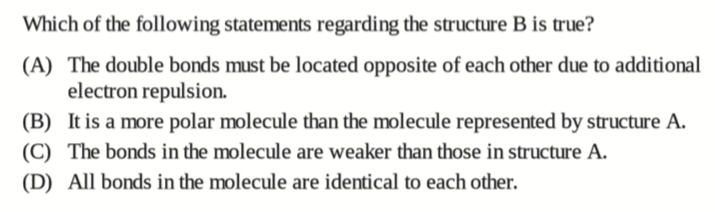• 对于共振结构，Lewis 结构式并不全面（甚至可以说是有缺陷的），实际上并没有两根键，而是这 $$2$$ 根键在 $$4$$ 个不同位置上做运动，所以每根键的键级和强度都是等价的

### Formal Charge 形式电荷

$\text{Formal Charge} = \text{(Valence Electrons)} - \text{(Unbonded Electrons)} - \text{(Bonds)}$

• 对于下图，我们可以分别计算 $$\ce{S}, \ce{O}$$ 的形式电荷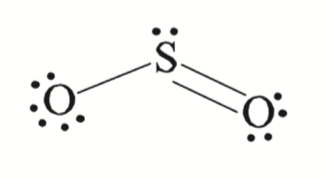• $$\ce{S}$$: 本身原有最外层电子为 $$6$$，在图中剩下的 Lone Pair 为，$$2$$，连了 $$3$$ 根键，所以 $$\text{Formal Charge} = 6 - 2 - 3 = 1$$

• 同理可以算出，$$\text{Left } \ce{O} = 6-6-1 = -1$$$$\text{Right } \ce{O} = 6-4-2 = 0$$

• 形式电荷为 $$0$$ 的时候是该物质最有可能的结构，如下图，更有可能的结构是 左边，而不是右边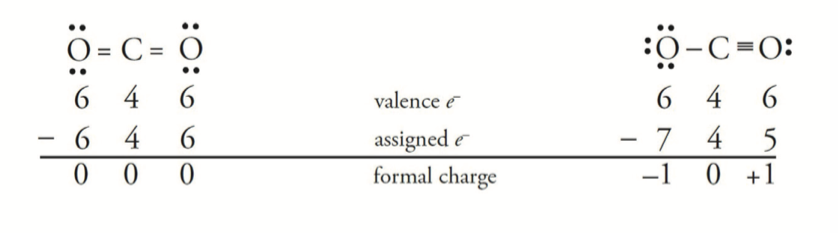• 这同样解释了为什么 元素周期表法 是绘制路易斯结构式较为有效的方法之一

• 对于无法计算为 $$0$$ 的结构，电负性更高的元素更有可能 Formal Charge 为负数，例如下图所示，我们认为图 3 是一个更有可能的选择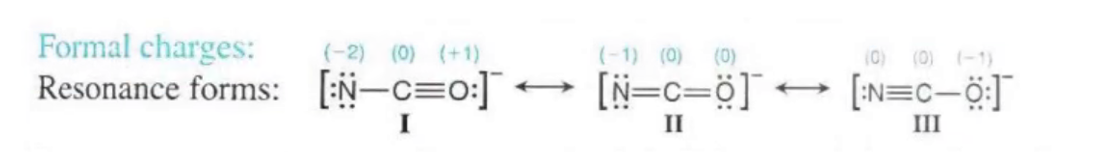• AP FRQ 2017: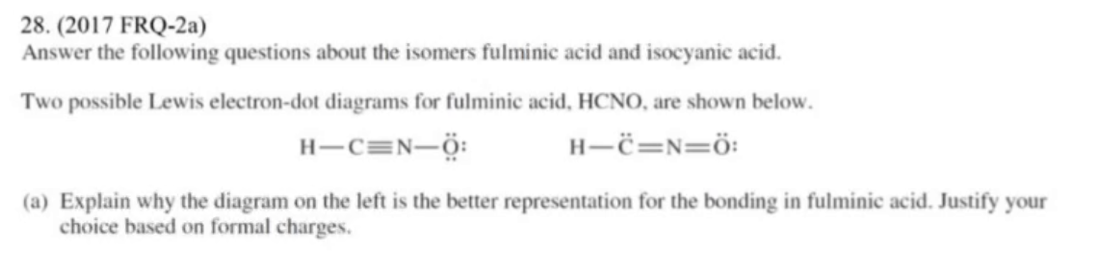Tips: Formal Charge 可以通过元素周期表法和键的数目快速计算，比如左图中 $$\ce{H}$$ 应该伸出 $$1$$ 根键，实际伸出 $$1$$ 根键，那么 $$H$$ 的 Formal Charge 便为 $$0$$。同理，对于 $$\ce{N}$$ 而言，应该伸出 $$3$$ 根键，实际伸出 $$4$$ 根键，那么 $$N$$ 的 Formal Charge 便为 $$+1$$。所以我们可以粗略认为，多伸键为正，少伸键为负

• 左边的结构 Formal Charge 依次为 $$\pu{0 0 +1 -1}$$，右边为 $$\pu{0 -1 +1 0}$$，而负 Formal Charge 一般在电负性高的元素上，所以我们认为，左边的结构更有可能（在实际考试中需写出 Formal Charge 计算过程）